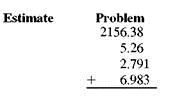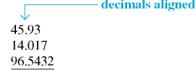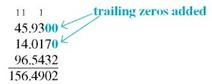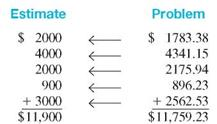# [Solved] First Use Front-End Rounding to Estimate and Then Find the Exact

Question 211
Essay

## First use front-end rounding to estimate and then find the exact answer. (See Examples.)Adding Decimal Numbers Add 45.93 + 14.017 + 96.5432. SOLUTION First, align place values by lining up the decimal points.Although not required, you can add trailing zeros if you wish so that each number has the same number of digits to the right of the decimal point. Then, add from right to left.Adding Dollars Exam and CentsDuring a recent week, a manager made the following bank deposits to a business account: \$1783.38, \$4341.15, \$2175.94, \$896.23, and \$2562.53. Use front-end rounding to estimate the total deposits and then find the total deposits. SOLUTIONThe total deposits for the week were \$11,759.23, which is close to our estimate.

10+ million students use Quizplus to study and prepare for their homework, quizzes and exams through 20m+ questions in 300k quizzes.

Explore our library and get Management Homework Help with various study sets and a huge amount of quizzes and questions

3.5K

Study sets

66.5K

Quizzes

5.9M

Questions

Upload material to get free accessInvite a friend and get free accessSubscribe and get an instant access